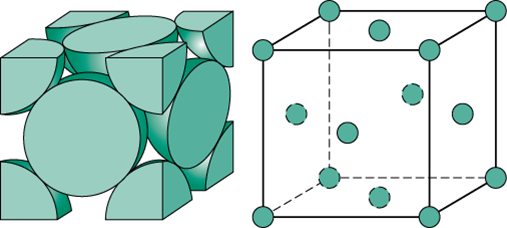Q

# Help me understand! Number of atoms in the unit cell of Na (BCC) and Mg (FCC) are respectively

Number of atoms in the unit cell of Na (BCC) and Mg (FCC) are respectively

• Option 1)

4 and 4

• Option 2)

2 and 4

• Option 3)

4 and 2

• Option 4)

1 and 1

201 Views

Number of atom in BCC unit cell =

Number of atom in FCC unit cell =

No. of atoms(z) for face centered unit cell -

Lattice points: at corners and face centers of unit cell.

For face centered cubic (FCC), z=4.

- whereinOption 1)

4 and 4

This is incorrect

Option 2)

2 and 4

This is correct

Option 3)

4 and 2

This is incorrect

Option 4)

1 and 1

This is incorrect

Exams
Articles
Questions